# NCERT solutions for Mathematics Exemplar Class 6 chapter 4 - Fractions and Decimals [Latest edition]

#### Chapters## Chapter 4: Fractions and Decimals

Exercise
Exercise [Pages 57 - 67]

### NCERT solutions for Mathematics Exemplar Class 6 Chapter 4 Fractions and Decimals Exercise [Pages 57 - 67]

#### Choose the correct alternative:

Exercise | Q 1 | Page 57

The fraction which is not equal to 4/5 is ______.

• 40/50

• 12/15

• 16/20

• 9/15

Exercise | Q 2 | Page 58

The two consecutive integers between which the fraction ______.

• 5 and 6

• 0 and 1

• 5 and 7

• 6 and 7

Exercise | Q 3 | Page 58

When 1/4 is written with denominator as 12, its numerator is ______.

• 3

• 8

• 24

• 12

Exercise | Q 4 | Page 58

Which of the following is not in the lowest form?

• 7/5

• 15/20

• 13/33

• 27/28

Exercise | Q 5 | Page 58

If 5/8 = 20/p, then value of p is ______.

• 23

• 2

• 32

• 16

Exercise | Q 6 | Page 58

Which of the following is not equal to the others?

• 6/8

• 12/16

• 15/25

• 18/24

Exercise | Q 7 | Page 58

Which of the following fractions is the greatest?

• 5/7

• 5/6

• 5/9

• 5/8

Exercise | Q 8 | Page 58

Which of the following fractions is the smallest?

• 7/8

• 9/8

• 3/8

• 5/8

Exercise | Q 9 | Page 58

Sum of 4/17 and 15/17 is ______.

• 19/17

• 11/17

• 19/34

• 2/17

Exercise | Q 10 | Page 58

On subtracting 5/9 from 19/9, the result is ______.

• 24/9

• 14/9

• 14/18

• 14/0

Exercise | Q 11 | Page 58

0.7499 lies between ______.

• 0.7 and 0.74

• 0.75 and 0.79

• 0.749 and 0.75

• 0.74992 and 0.75

Exercise | Q 12 | Page 59

0. 023 lies between ______.

• 0.2 and 0.3

• 0.02 and 0.03

• 0.03 and 0.029

• 0.026 and 0.024

Exercise | Q 13 | Page 59

11/7 can be expressed in the form ______.

• 7 1/4

• 4 1/7

• 1 4/7

• 11 1/7

Exercise | Q 14 | Page 59

The mixed fraction 5 4/7 can be expressed as ______.

• 33/7

• 39/7

• 33/4

• 39/4

Exercise | Q 15 | Page 59

0.07 + 0.008 is equal to ______.

• 0.15

• 0.015

• 0.078

• 0.78

Exercise | Q 16 | Page 59

Which of the following decimals is the greatest?

• 0.182

• 0.0925

• 0.29

• 0.038

Exercise | Q 17 | Page 59

Which of the following decimals is the smallest?

• 0.27

• 1.5

• 0.082

• 0.103

Exercise | Q 18 | Page 59

13.572 correct to the tenths place is ______.

• 10

• 13.57

• 14.5

• 13.6

Exercise | Q 19 | Page 59

15.8 – 6.73 is equal to ______.

• 8.07

• 9.07

• 9.13

• 9.25

Exercise | Q 20 | Page 59

The decimal 0.238 is equal to the fraction ______.

• 119/500

• 238/25

• 119/25

• 119/50

#### Fill in the blank:

Exercise | Q 21 | Page 59

A number representing a part of a ______ is called a fraction.

Exercise | Q 22 | Page 59

Fractions with the same denominator are called ______ fractions.

Exercise | Q 23 | Page 59

Fractions with the same denominator are called ______ fractions.

Exercise | Q 24 | Page 59

13 5/8 is a ______ fraction.

Exercise | Q 25 | Page 60

18/5 is an ______ fraction.

Exercise | Q 26 | Page 60

7/19 is a ______ fraction.

Exercise | Q 27 | Page 60

5/8 and 3/8 are ______ proper fraction.

Exercise | Q 28 | Page 60

6/11 and 6/13 are ______ proper fractions.

Exercise | Q 29 | Page 60

The fraction 6/15 in simplest form is ______.

Exercise | Q 30 | Page 60

The fraction 17/34 in simplest form is ______.

Exercise | Q 31 | Page 60

18/135 and 90/675 are proper, unlike and ______ fractions.

Exercise | Q 32 | Page 60

8 2/7 is equal to the improper fraction ______.

Exercise | Q 33 | Page 60

87/7 is equal to the mixed fraction ______.

Exercise | Q 34 | Page 60

9 + 2/10 + 6/100 is equal to the decimal number ______.

Exercise | Q 35 | Page 60

Decimal 16.25 is equal to the fraction ______.

Exercise | Q 36 | Page 60

Decimal 16.25 is equal to the fraction ______.

Exercise | Q 37 | Page 60

17/9 + 41/9 = ______.

Exercise | Q 38 | Page 60

67/14 - 24/14 = ______.

Exercise | Q 39 | Page 60

17/2 + 3 1/2 = ______.

Exercise | Q 40 | Page 60

9 1/4 - 5/4 = ______.

Exercise | Q 41 | Page 60

4.55 + 9.73 = ______.

Exercise | Q 42 | Page 60

8.76 – 2.68 = ______.

Exercise | Q 43 | Page 60

The value of 50 coins of 50 paisa = Rs ______.

Exercise | Q 44 | Page 60

3 Hundredths + 3 tenths = ______.

#### State whether the following statement is True or False:

Exercise | Q 45 | Page 60

Fractions with same numerator are called like fractions.

• True

• False

Exercise | Q 46 | Page 60

Fraction 18/39 is in its lowest form.

• True

• False

Exercise | Q 47 | Page 61

Fractions 15/39 and 45/117 are equivalent fractions.

• True

• False

Exercise | Q 48 | Page 61

The sum of two fractions is always a fraction.

• True

• False

Exercise | Q 49 | Page 61

The result obtained by subtracting a fraction from another fraction is necessarily a fraction.

• True

• False

Exercise | Q 50 | Page 61

If a whole or an object is divided into a number of equal parts, then each part represents a fraction.

• True

• False

Exercise | Q 51 | Page 61

The place value of a digit at the tenths place is 10 times the same digit at the ones place.

• True

• False

Exercise | Q 52 | Page 61

The place value of a digit at the hundredths place is 1/10 times the same digit at the tenths place.

• True

• False

Exercise | Q 53 | Page 61

The decimal 3.725 is equal to 3.72 correct to two decimal places.

• True

• False

Exercise | Q 54 | Page 61

In the decimal form, fraction 25/8 = 3.125.

• True

• False

Exercise | Q 55 | Page 61

The decimal 23.2 = 23 2/5

• True

• False

Exercise | Q 56 | Page 61

The fraction represented by the shaded portion in the adjoining figure is ______.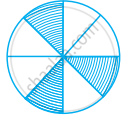• True

• False

Exercise | Q 57 | Page 61

The fraction represented by the unshaded portion in the adjoining figure is 5/9.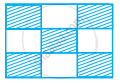• True

• False

Exercise | Q 58 | Page 61

25/19 + 6/19 = 31/38

• True

• False

Exercise | Q 59 | Page 61

8/18 - 8/15 = 8/3

• True

• False

Exercise | Q 60 | Page 61

7/12 + 11/12 = 3/2

• True

• False

Exercise | Q 61 | Page 61

3.03 + 0.016 =3.019

• True

• False

Exercise | Q 62 | Page 61

42.28 – 3.19 = 39.09

• True

• False

Exercise | Q 63 | Page 61

16/25 > 13/25

• True

• False

Exercise | Q 64 | Page 61

19.25 < 19.053

• True

• False

Exercise | Q 65 | Page 61

13.730 = 13.73

• True

• False

#### Fill in the blanks using ‘>’, ‘<’ or ‘=’ :

Exercise | Q 66 | Page 61

11/16 ______ 14/15

Exercise | Q 67 | Page 61

8/15 ______ 95/14

Exercise | Q 68 | Page 62

12/75 ______ 32/200

Exercise | Q 69 | Page 62

3.25 ______ 3.4

Exercise | Q 70 | Page 62

18/15 ______ 1.3

Exercise | Q 71 | Page 62

6.25 ______ 25/4

Exercise | Q 72 | Page 62

Write the fraction represented by the shaded portion of the adjoining figure: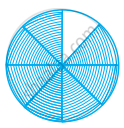Exercise | Q 73 | Page 62

Write the fraction represented by the unshaded portion of the adjoining figure: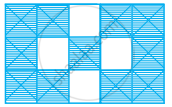Exercise | Q 74 | Page 62

Ali divided one fruit cake equally among six persons. What part of the cake he gave to each person?

Exercise | Q 75 | Page 62

Arrange 12.142, 12.124, 12.104, 12.401 and 12.214 in ascending order.

Exercise | Q 76 | Page 62

Write the largest four digit decimal number less than1using the digits 1, 5, 3 and 8 once.

Exercise | Q 77 | Page 62

Using the digits 2, 4, 5 and 3 once, write the smallest four digit decimal number.

Exercise | Q 78 | Page 62

Express 11/20 as a decimal.

Exercise | Q 79 | Page 62

Express 6 2/3 as an improper fraction.

Exercise | Q 80 | Page 62

Express 3 2/5 as a decimal.

Exercise | Q 81 | Page 62

Express 0.041 as a fraction.

Exercise | Q 82 | Page 62

Express 6.03 as a mixed fraction.

Exercise | Q 83 | Page 62

Convert 5201g to kg.

Exercise | Q 84 | Page 63

Convert 2009 paise to rupees and express the result as a mixed fraction.

Exercise | Q 85 | Page 63

Convert 1537cm to m and express the result as an improper fraction.

Exercise | Q 86 | Page 63

Convert 2435m to km and express the result as mixed fraction.

Exercise | Q 87 | Page 63

Arrange the fractions 2/3, 3/4, 1/2 and 5/6 in ascending order.

Exercise | Q 88 | Page 63

Arrange the fractions 6/7, 7/8, 4/5 and 3/4 in descending order.

Exercise | Q 89 | Page 63

Write 3/4 as a fraction with denominator 44.

Exercise | Q 90 | Page 63

Write 5/6 as a fraction with numerator 60.

Exercise | Q 91 | Page 63

Write 129/8 as a mixed fraction.

Exercise | Q 92 | Page 63

Round off 20.83 to nearest tenths.

Exercise | Q 93 | Page 63

Round off 75.195 to nearest hundredths.

Exercise | Q 94 | Page 63

Round off 27.981 to nearest tenths.

Exercise | Q 95 | Page 63

Add the fractions 3/8 and 2/3.

Exercise | Q 96 | Page 63

Add the fractions 3/8 and 6 3/4.

Exercise | Q 97 | Page 63

Subtract 1/6 from 1/2.

Exercise | Q 98 | Page 63

Subtract 8 1/3 from 100/9.

Exercise | Q 99 | Page 63

Subtract 1 1/4 and 6 1/2.

Exercise | Q 100 | Page 63

Add 1 1/4 and 6 1/2.

Exercise | Q 101 | Page 63

Katrina rode her bicycle 6 1/2 km in the morning and 8 3/4 km in the evening. Find the distance travelled by her altogether on that day.

Exercise | Q 102 | Page 64

A rectangle is divided into certain number of equal parts. If 16 of the parts so formed represent the fraction 1/4. find the number of parts in which the rectangle has been divided.

Exercise | Q 103 | Page 64

Grip size of a tennis racquet is 11 9/80 cm. Express the size as an improper fraction.

Exercise | Q 104 | Page 64

On an average 1/10 of the food eaten is turned into organism’s own body and is available for the nextlevel of consumer in a food chain. What fraction of the food eaten is not available for the next level?

Exercise | Q 105 | Page 64

Mr. Rajan got a job at the age of 24 years and he got retired from the job at the age of 60 years. What fraction of his age till retirement was he in the job?

Exercise | Q 106 | Page 64

The food we eat remains in the stomach for a maximum of 4 hours. For what fraction of a day, does it remain there?

Exercise | Q 107 | Page 64

What should be added to 25.5 to get 50?

Exercise | Q 108 | Page 64

Alok purchased 1 kg 200 g potatoes, 250 g dhania, 5 kg 300 g onion, 500 g palak and 2 kg 600 g tomatoes. Find the total weight of his purchases in kilograms.

Exercise | Q 109 | Page 64

Arrange in ascending order:

0.011, 1.001, 0.101, 0.110

Exercise | Q 110 | Page 64

20.02 and 2.002

Exercise | Q 111.(i) | Page 64

It was estimated that because of people switching to Metro trains, about 33000 tonnes of CNG, 3300 tonnes of diesel and 21000 tonnes of petrol was saved by the end of year 2007. Find the fraction of the quantity of diesel saved to the quantity of petrol saved.

Exercise | Q 111.(ii) | Page 64

It was estimated that because of people switching to Metro trains, about 33000 tonnes of CNG, 3300 tonnes of diesel and 21000 tonnes of petrol was saved by the end of year 2007. Find the fraction of the quantity of diesel saved to the quantity of CNG saved.

Exercise | Q 112 | Page 65

Energy content of different foods are as follows:

 Food Energy Content per kg. Wheat 3.2 Joules Rice 5.3 Joules Potatoes (Cooked) 3.7 Joules Milk 3.0 Joules

Which food provides the least energy and which provides the maximum?
Express the least energy as a fraction of the maximum energy.

Exercise | Q 113 | Page 65

A cup is 1/3 full of milk. What part of the cup is still to be filled by milk to make it full?

Exercise | Q 114 | Page 65

Mary bought 3 1/2 m of lace. She used 1 3/4 m of lace for her new dress. How much lace is left with her?

Exercise | Q 115 | Page 65

When Sunita weighed herself on Monday, she found that she had gained 1 1/4 5 kg. Earlier her weight was 46 3/8 kg. What was her weight on Monday?

Exercise | Q 116 | Page 65

Sunil purchased 12 1/2 litres of juice on Monday and 14 3/4 litres of juice on Tuesday. How many litres of juice did he purchase together in two days?

Exercise | Q 117 | Page 65

Nazima gave 2 3/4 litres out of the 5 1/2 litres of juice she purchased to her friends. How many litres of juice is left with her?

Exercise | Q 118 | Page 65

Roma gave a wooden board of length 150 1/4 cm to a carpenter for making a shelf. The Carpenter sawed off a piece of 40 1/5 cm from it. What is the length of the remaining piece?

Exercise | Q 119 | Page 66

Nasir travelled 3 1/2 km in a bus and then walked 1 1/8 km to reach a town. How much did he travel to reach the town?

Exercise | Q 120 | Page 66

The fish caught by Neetu was of weight 3 3/4 kg and the fish caught by Narendra was of weight 2 1/2 kg. How much more did Neetu’s fish weigh than that of Narendra?

Exercise | Q 121 | Page 66

Neelam’s father needs 1 3/4 m of cloth for the skirt of Neelam’s new dress and 1/2 m for the scarf. How much cloth must he buy in all?

Exercise | Q 122.(a) | Page 66

What is wrong in the following additions?

8 1/2 = 8 2/4

+ 4 1/4 = 4 1/4

= 12 3/8

Exercise | Q 122.(b) | Page 66

What is wrong in the following additions?

6 1/2

+ 2 1/4

= 8 2/6 = 8 1/3

Exercise | Q 123 | Page 66

Which one is greater?

1 metre 40 centimetres + 60 centimetres or 2.6 metres.

#### Match the fractions of Column I with the shaded or marked portion of figures of Column II:

Exercise | Q 124 | Page 66
 Column I Column II (i) 6/4 (A)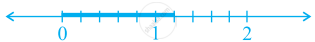(ii) 6/10 (B)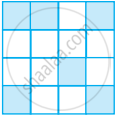(iii) 6/6 (C)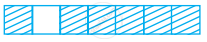(iv) 6/16 (D)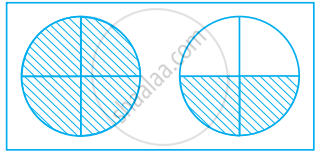(v) 6/7 (E)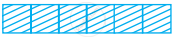Exercise | Q 125 | Page 67

Find the fraction that represents the number of natural numbers to total numbers in the collection 0, 1, 2, 3, 4, 5. What fraction will it be for whole numbers?

Exercise | Q 126 | Page 67

Write the fraction representing the total number of natural numbers in the collection of numbers –3, – 2, –1, 0, 1, 2, 3. What fraction will it be for whole numbers? What fraction will it be for integers?

Exercise | Q 127 | Page 67

Write a pair of fractions whose sum is 7/11 and difference is 2/11.

Exercise | Q 128 | Page 67

What fraction of a straight angle is a right angle?

Exercise | Q 129.(i) | Page 67

Put the card in the right bag.

Card - 3/7

•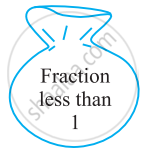•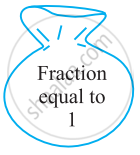•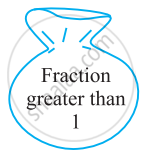Exercise | Q 129.(ii) | Page 67

Put the card in the right bag.

Card - 4/4

•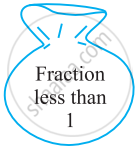•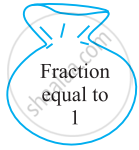•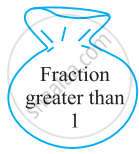Exercise | Q 129.(iii) | Page 67

Put the card in the right bag.

Card - 9/8

•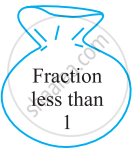••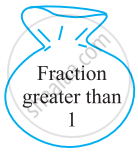Exercise | Q 129.(iv) | Page 67

Put the card in the right bag.

Card - 8/9

•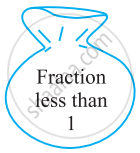•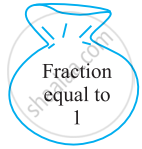•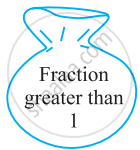Exercise | Q 129.(v) | Page 67

Put the card in the right bag.

Card - 5/6

•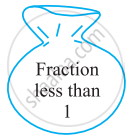•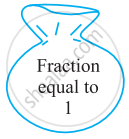•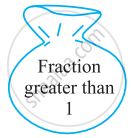Exercise | Q 129.(vi) | Page 67

Put the card in the right bag.

Card - 6/11

•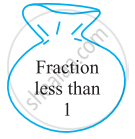•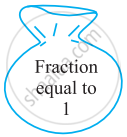•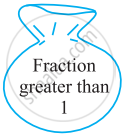Exercise | Q 129.(vii) | Page 67

Put the card in the right bag.

Card - 18/18

••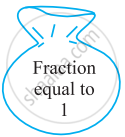•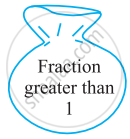Exercise | Q 129.(viii) | Page 67

Put the card in the right bag.

Card - 19/25

•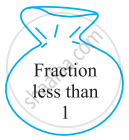••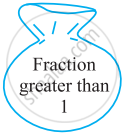Exercise | Q 129.(ix) | Page 67

Put the card in the right bag.

Card - 2/3

•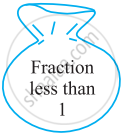•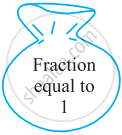•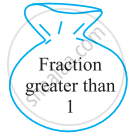Exercise | Q 129.(x) | Page 67

Put the card in the right bag.

Card - 13/17

•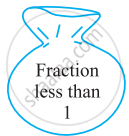•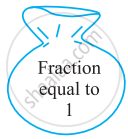•## Chapter 4: Fractions and Decimals

Exercise## NCERT solutions for Mathematics Exemplar Class 6 chapter 4 - Fractions and Decimals

NCERT solutions for Mathematics Exemplar Class 6 chapter 4 (Fractions and Decimals) include all questions with solution and detail explanation. This will clear students doubts about any question and improve application skills while preparing for board exams. The detailed, step-by-step solutions will help you understand the concepts better and clear your confusions, if any. Shaalaa.com has the CBSE Mathematics Exemplar Class 6 solutions in a manner that help students grasp basic concepts better and faster.

Further, we at Shaalaa.com provide such solutions so that students can prepare for written exams. NCERT textbook solutions can be a core help for self-study and acts as a perfect self-help guidance for students.

Concepts covered in Mathematics Exemplar Class 6 chapter 4 Fractions and Decimals are Concept of Fractions, Fraction on the Number Line, Types of Fraction, Concept of Proper Fractions, Improper Fraction and Mixed Fraction, Concept for Equivalent Fractions, Like and Unlike Fraction, Simplest Form of a Fraction, Comparing Fractions, Addition of Fraction, Subtraction of Fraction, Concept of Decimal Numbers, Place Value in the Context of Decimal Fraction, Concept of Tenths, Hundredths and Thousandths in Decimal, Representing Decimals on the Number Line, Interconversion of Fraction and Decimal, Comparing Decimal Numbers, Using Decimal Number as Units, Addition of Decimal Numbers, Subtraction of Decimals Fraction.

Using NCERT Class 6 solutions Fractions and Decimals exercise by students are an easy way to prepare for the exams, as they involve solutions arranged chapter-wise also page wise. The questions involved in NCERT Solutions are important questions that can be asked in the final exam. Maximum students of CBSE Class 6 prefer NCERT Textbook Solutions to score more in exam.

Get the free view of chapter 4 Fractions and Decimals Class 6 extra questions for Mathematics Exemplar Class 6 and can use Shaalaa.com to keep it handy for your exam preparation